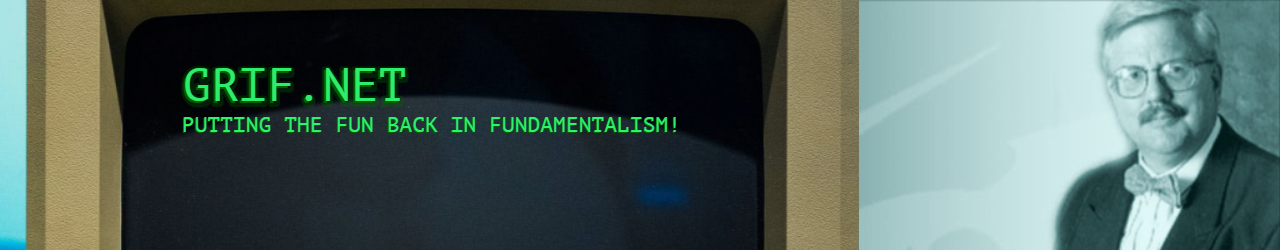# 08/07/17 Grif.Net – I Now Know

## 08/07/17 Grif.Net – I Now Know

I now know . . =
.

. . . that Chicago Bears fans never change lightbulbs since =
they=E2=80=99re content to live in the shadow of the Packers and =
Vikings.

. . . that since my wife and I can’t stand each other’s =
family, we must be kin dread souls.

. . . that =
facts about subtraction just don’t add up.

. . . that a =
Cajun breakfast has to consist of more than blackened =
pop-tarts.

. . . that the =
gym always seems hotter after the game when the fans are =
gone.

. . . that =
being born free doesn=E2=80=99t stop the government from taxing you to =
death.

. . . that the =
more liberals I meet, the more I like my dog.

. . . that I =
didn=E2=80=99t fight my way to the top of the food chain to become a =
vegetarian.

. . . that I =
should have been better to my kids, since they will choose my nursing =
home.

. . . that you =
have to make the right choice now if you want the smoking or no smoking =
section in eternity.

. . . that I =
don=E2=80=99t need to pray =E2=80=9CLead me not into temptation=E2=80=9D =
since I seem to be able to find it for myself.

~~

Suggestions =
for changing the motto of the Grif.Net blog:

Way=
ne R said, "The Grif Net:  Stirred, not shaken … We all =
need a little Bond in our life!"

Dan P =
suggested =E2=80=9CThe Grif Net:  The fundament of good =
humor!=E2=80=9D=C2=A0 (Sadly, this would take a LOT of explanation, =
since foundament =3D=C2=A0 the butt of the joke. Defined as: Foundation or basis of something, including a =
person’s buttocks.)

Tom=
S said, =E2=80=9CStick with the =
original=E2=80=9D

M=
arilyn T opined, =E2=80=9CThe Grif Net: For those who were weaned on =
dill pickle juice=E2=80=9D

J=
im W thought "Making Calvinists Humorous Since 1996" sounded =
good

W=
illiam N said =E2=80=9CThe Grif Net
: Great Gift =
for Laughs and Groans=E2=80=9D

Oliver said =
just use the catch-word =E2=80=9CWitty=E2=80=9D.=C2=A0 =

Ken M twisted =
our original with =E2=80=9CPut the MENTAL back in =
FundaMENTAL=E2=80=9D.=C2=A0 That hurt.

😊 =

~~

Dr Bob Griffin =

bob@grif.net =
www.grif.net

"Jesus =
Knows Me, This I Love!"